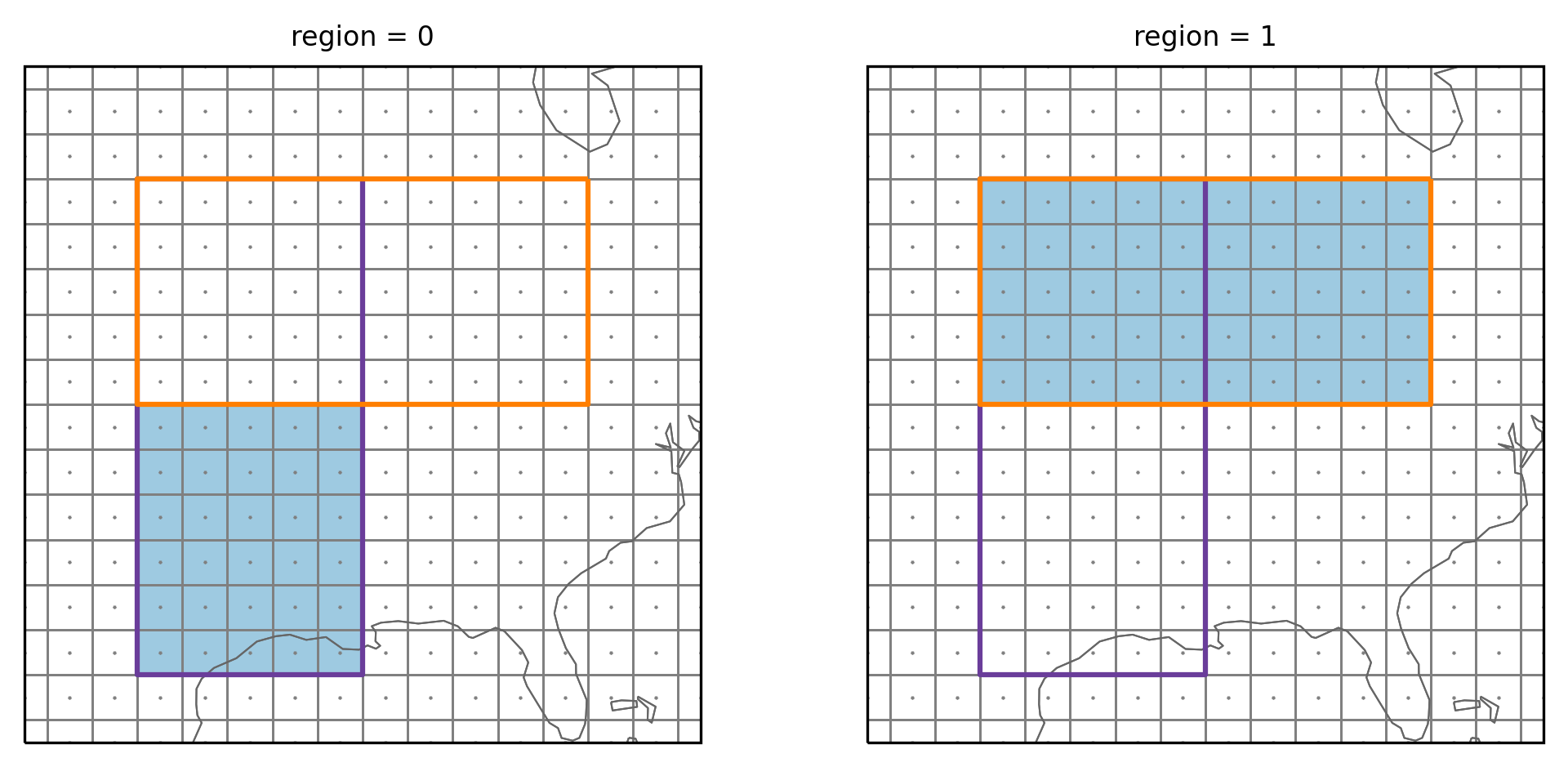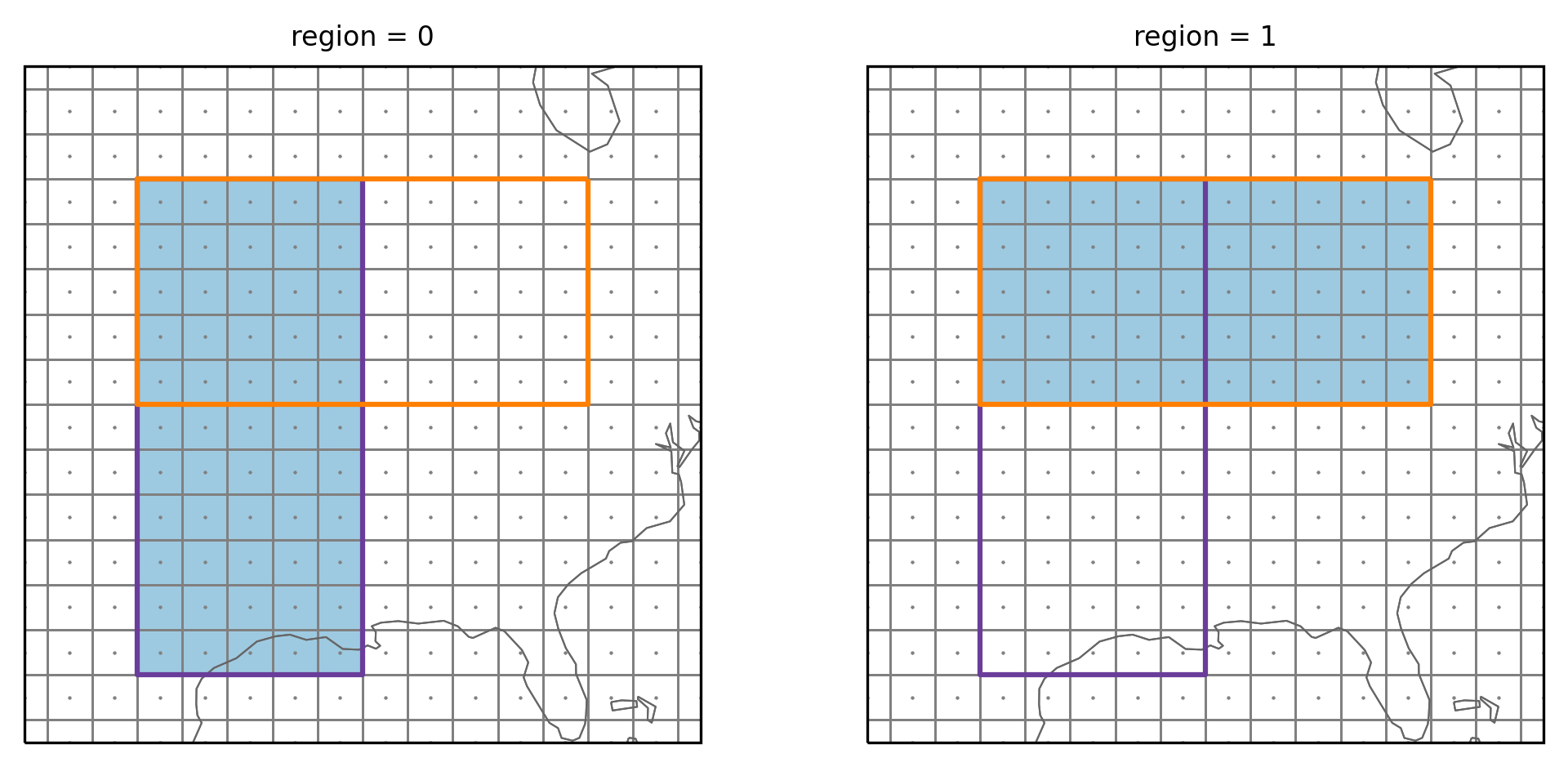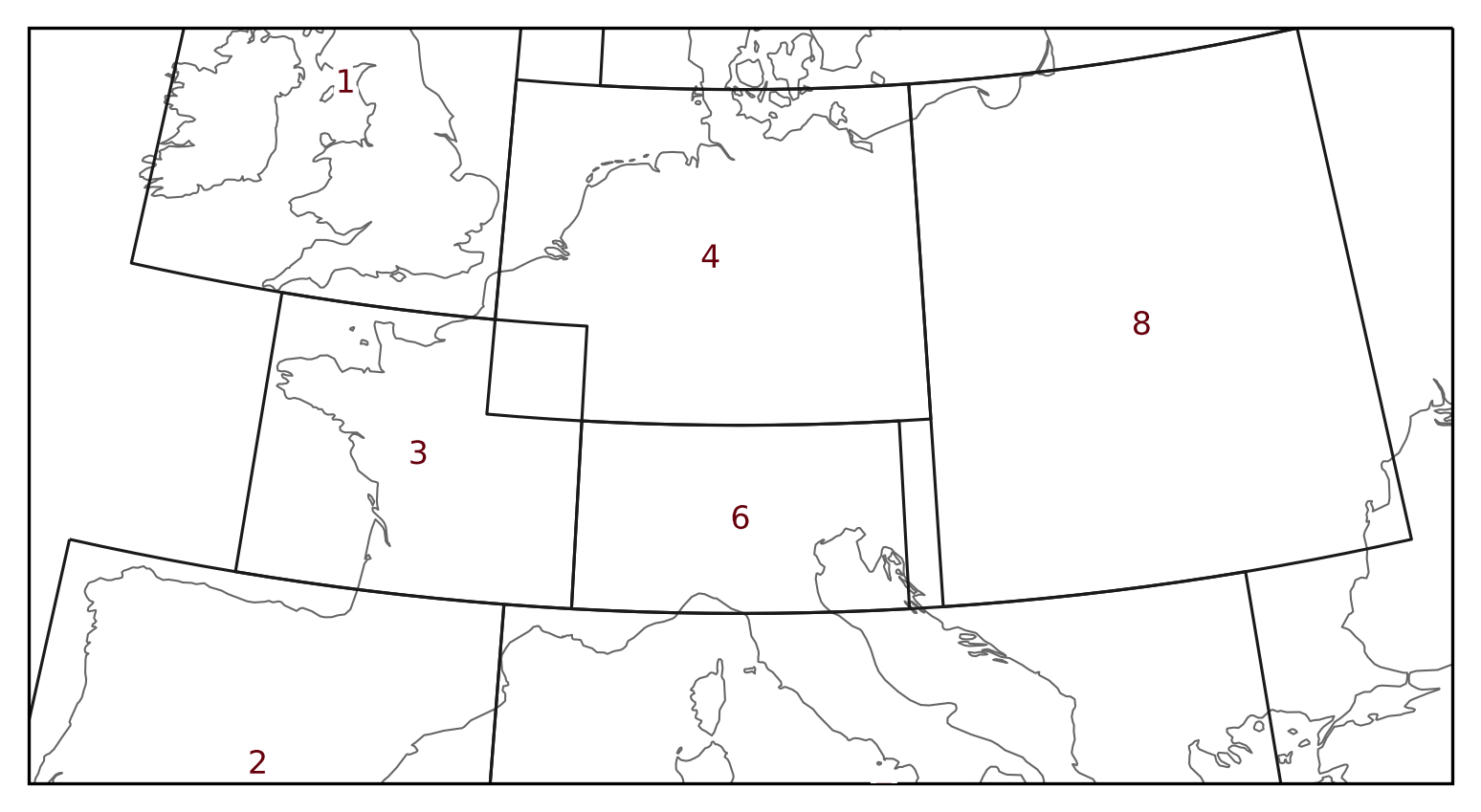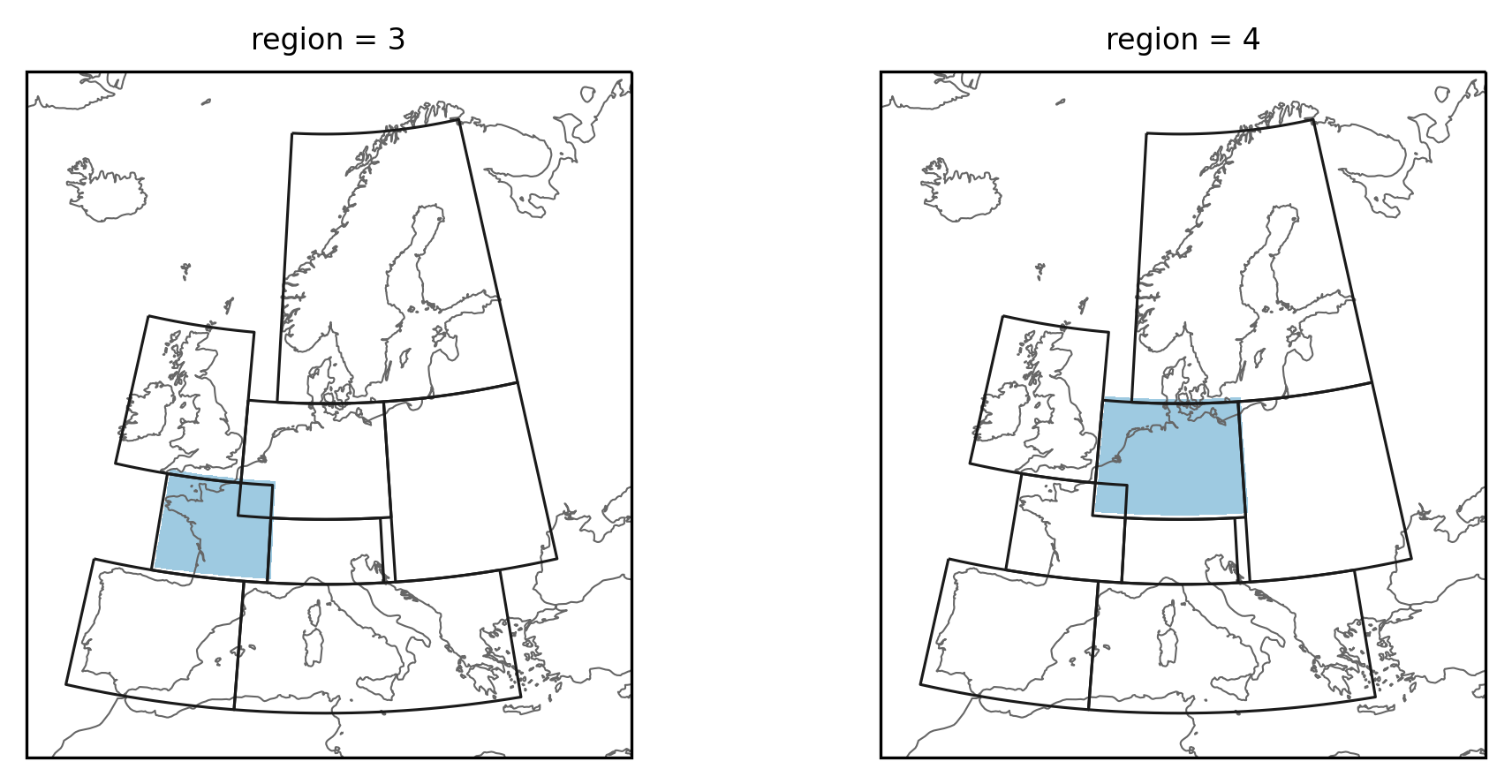None

Note

This tutorial was generated from an IPython notebook that can be accessed from github.

# Overlapping regions

Two or more on regions can share the same area - they overlap, as for example region 3 and 4 of the PRUDENCE regions This notebook illustrates how overlapping regions can be treated in regionmask.

## In short

Warning

Per default regionmask assumes regions are not overlapping!

Thus, when creating your own `Regions` you need to tell regionmask if they are overlapping.

```region = regionmask.Regions(..., overlap=True)
```

If you have two overlapping regions and `overlap=False` regionmask will silently assign the gridpoints of the overlapping regions to the one with the higher number, e.g., region 4 for PRUDENCE (this may change in a future version).

Note that `overlap` is correctly defined in `regionmask.defined_regions`.

## Example

To illustrate the problem we construct two regions in North America that partially overlap. One is horizontal, the other vertical.

Preparation

Import regionmask and check the version:

```import regionmask

```
```'0.10.0'
```

Other imports

```import xarray as xr
import numpy as np

import cartopy.crs as ccrs
import matplotlib.pyplot as plt

from matplotlib import colors as mplc
from shapely.geometry import Polygon

import matplotlib.patheffects as pe
```

Define some colors:

```cmap = mplc.ListedColormap(["none", "#9ecae1"])
```

Define helper function:

```def plot_region_vh(mask):

subplot_kws=dict(projection=ccrs.PlateCarree()),
col="region",
cmap=cmap,
transform=ccrs.PlateCarree(),
ec="0.5",
lw=0.25,
)

for ax in fg.axes.flatten():

ax.set_extent([-105, -75, 25, 55], ccrs.PlateCarree())
ax.plot(
ds_US.LON, ds_US.lat, "*", color="0.5", ms=0.5, transform=ccrs.PlateCarree()
)
```

Define the polygons:

```coords_v = np.array([[-90.0, 50.0], [-90.0, 28.0], [-100.0, 28.0], [-100.0, 50.0]])
coords_h = np.array([[-80.0, 50.0], [-80.0, 40.0], [-100.0, 40.0], [-100.0, 50.0]])
```

### Default behavior (`overlap=False`)

Fe first test what happens if we keep the default value of `overlap=False`:

```region_vh = regionmask.Regions([coords_v, coords_h])
```

```ds_US = regionmask.core.utils.create_lon_lat_dataarray_from_bounds(
*(-160, -29, 2), *(76, 13, -2)
)

```

```plot_region_vh(mask_vh)
```The small gray points show the gridpoint center and the vertical and horizontal lines are the gridpoint boundaries. The colored rectangles are the two regions. The vertical region has the number 1 and the horizontal region the number 2. We can see that only the gridpoints in the lower part of the vertical (magenta) region were assigned to it. All gridpoints of the overlapping part are now assigned to the horizontal (orange) region. As mentioned the gridpoints are assigned to the region with the higher number By switching the order of the regions you could have the common gridpoints assigned to the vertical region.

### Setting `overlap=True`

As mentioned regionmask assumes regions are not overlapping, so you need to pass `overlap=True` to the constructor:

```region_overlap = regionmask.Regions([coords_v, coords_h], overlap=True)

region_overlap
```
```<regionmask.Regions 'unnamed'>
overlap:  True

Regions:
0 r0 Region0
1 r1 Region1

[2 regions]
```

Now it says `overlap:  True` - and we can again create a mask:

```mask_overlap = region_overlap.mask_3D(ds_US)
```

and plot it

```plot_region_vh(mask_overlap)
```Now the gridpoints in the overlapping part are assigned to both regions.

## PRUDENCE regions

The PRUDENCE regions are a real-world example of overlapping areas. The prudence regions already set `overlap=True`.

```prudence = regionmask.defined_regions.prudence
prudence
```
```<regionmask.Regions 'PRUDENCE'>
Source:   Christensen and Christensen, 2007, Climatic Change 81:7-30 (https:/...
overlap:  True

Regions:
1 BI     British Isles
2 IP Iberian Peninsula
3 FR            France
4 ME        Mid-Europe
5 SC       Scandinavia
6 AL              Alps
7 MD     Mediterranean
8 EA    Eastern Europe

[8 regions]
```

Regions 3 and 4 overlap in Western France:

```proj = ccrs.LambertConformal(central_longitude=10)

text_kws = dict(
bbox=dict(color="none"),
path_effects=[pe.withStroke(linewidth=3, foreground="w")],
color="#67000d",
)

ax = prudence.plot(
projection=proj, text_kws=text_kws, resolution="50m", line_kws=dict(lw=0.75)
)

ax.set_extent([-10.0, 30.0, 40.0, 55.0], ccrs.PlateCarree())
```### Create mask of PRUDENCE regions

```lon = np.arange(-12, 33, 0.5)
lat = np.arange(72, 33, -0.5)

```
```proj = ccrs.LambertConformal(central_longitude=10)

subplot_kws=dict(projection=proj),
col="region",
cmap=cmap,